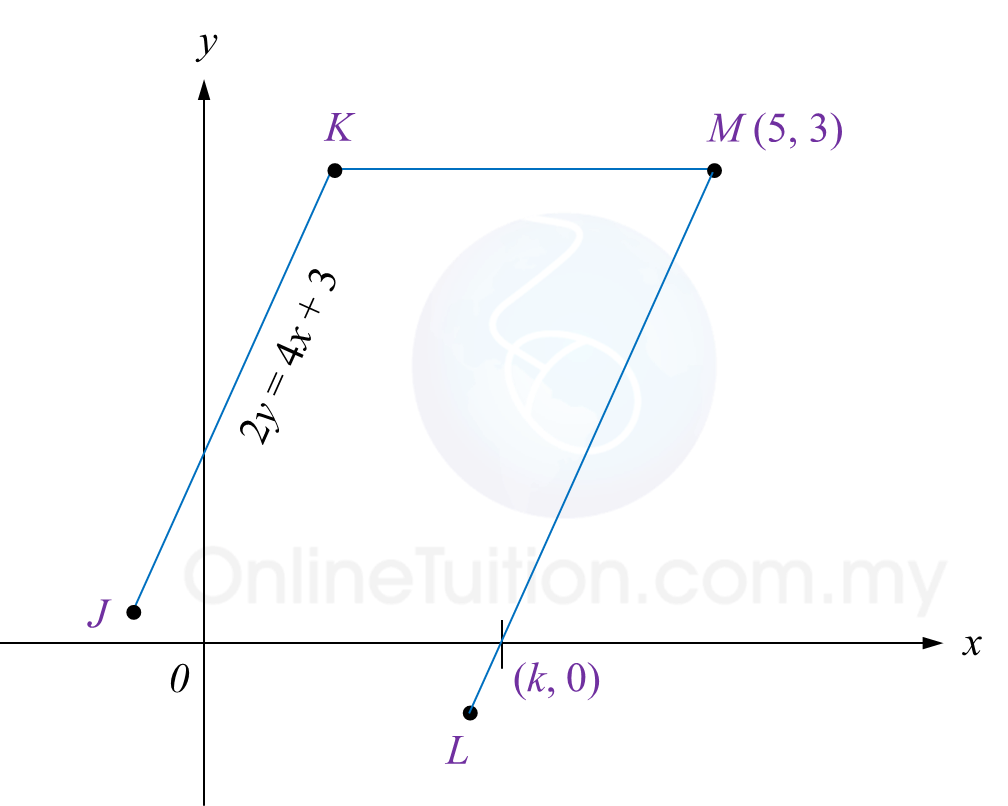# SPM Mathematics 2018, Paper 2 (Questions 7 & 8)

Question 7 (6 marks):
Diagram 6 shows two parallel straight lines, JK and LM, drawn on a Cartesian plane.
The straight line KM is parallel to the x-axis.Diagram 6

Find
(a) the equation of the straight line KM,
(b) the equation of the straight line LM,
(c) the value of k.

Solution:
(a)

The equation of the straight line KM is y = 3

(b)

(c)

Question 8 (6 marks):

(a) Find the value of m and of n if AB = I.
(b) Write the following simultaneous linear equation as matrix equation:
4x – 2y = 3
3xy = 2
Hence, by using matrix method, calculate the value of x and of y.

Solution:
(a)

(b)